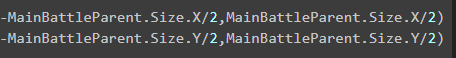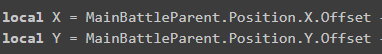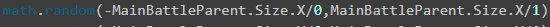# Trying to make symbols spawn in a random position inside a frame

Hello, So lately I have been working on a game and I am now working on a section for it.
It will spawn 3 random symbols with a random position inside a frame.

Here is the script I got so far.

``````		for i, z in pairs(SymbolFolder:GetChildren()) do

local X = MainBattleParent.Position.X + math.random(-MainBattleParent.Size.X/2,MainBattleParent.Size.X/2)
local Y = MainBattleParent.Position.Y + math.random(-MainBattleParent.Size.Y/2,MainBattleParent.Size.Y/2)

z.Position = Vector3.new(X,Y,0)
z.Visible = true
end
``````

When I test the game I get this error.

``````attempt to perform arithmetic (div) on UDim and number
``````

Many thanks for any help.

2 Likes

When defining ‘X’ or ‘Y’ as a property of a size or position, you are defining the UDim (scale,offset). Depending on if you are using scale or offset, add that to X and Y. e.g (Size.X.Scale). also, you are setting the position of a UI with a `Vector3` value, it should be `UDim2.new()`.

1 Like

I tried applying what you said and I still am getting the same error.
If it’s not a big ask but can you show me how you would apply it to my script?

Are you using scale or offset?

1 Like

Offset.

All I’m changing is the position of the symbols.

Okay, so replace all the Position.X and Position.Y’s with Position.X.Offset and Position.Y.Offset.

Then when you set the Position, do `UDim2.fromOffset(X,Y)`

1 Like

It did something but I am still getting the same error.

Here is the script I got now.

``````		for i, z in pairs(SymbolFolder:GetChildren()) do

local X = MainBattleParent.Position.X.Offset + math.random(-MainBattleParent.Size.X/2,MainBattleParent.Size.X/2)
local Y = MainBattleParent.Position.Y.Offset + math.random(-MainBattleParent.Size.Y/2,MainBattleParent.Size.Y/2)

z.Position = UDim2.fromOffset(X,Y)
z.Visible = true
end
``````

do the same here,Size.X.Offset
Size.Y.Offset

1 Like

Ok the symbols move position and show just sometimes they appear out of the frame.

This is because of Offset. Offset is the position on your screen in pixels. If you wanted it inside the frame, you can consider using math.clamp() with the AbosluteSize or use Scale.

1 Like

How would I apply those to my script?remove thisthen change the both 1st arguments of math.random to 0, then the both 2nd arguments of math.random to 1then change this to fromScale

I can’t seem to fix it by adding this I just get the same error as before.

Show the script.

``````		for i, z in pairs(SymbolFolder:GetChildren()) do

local X = math.random(-MainBattleParent.Size.X/0,MainBattleParent.Size.X/1)
local Y = math.random(-MainBattleParent.Size.Y/0,MainBattleParent.Size.Y/1)

z.Position = UDim2.fromScale(X,Y)
z.Visible = true
end
``````No, I mean change both of the arguments to use 0 and 1. e.g math.random(0,1).

This works just only makes them spawn in the corners of the frame.

That’s because 0,0 starts at the top left corner.

Instead of using offset, just use scale, and use `random.new()` as the scale values.

``````local random1 = random.new()
local random2 = random.new()
for i, z in pairs(SymbolFolder:GetChildren()) do
random1:NextNumber()
random2:NextNumber()

z.Position = Udim2.new(random1,0,random2,0)
z.Visible = true
end
``````

Sadly not they all still spawn in the cornors.